# Iterative Calculation in Seeq Data Lab

## Recommended Posts

A few weeks ago in Office Hours, a Seeq user asked how to perform iterative calculation in which the next value of the calculation relies on its previous values. This functionality is currently logged as a feature request. In the meantime, users can utilize Seeq Data Lab and push the calculated result from Seeq Data Lab to Seeq Workbench. Let's check out the example below:

There are a total of 4 signals, Signal G, Signal H, Signal J, and Signal Y added to Seeq workbench. The aim is to calculate the value of Signal Y, under the selected
period.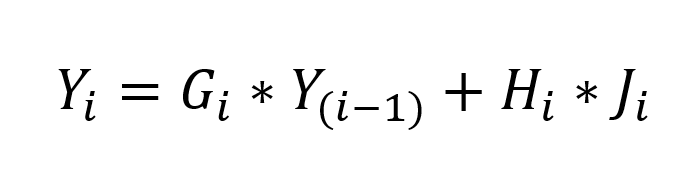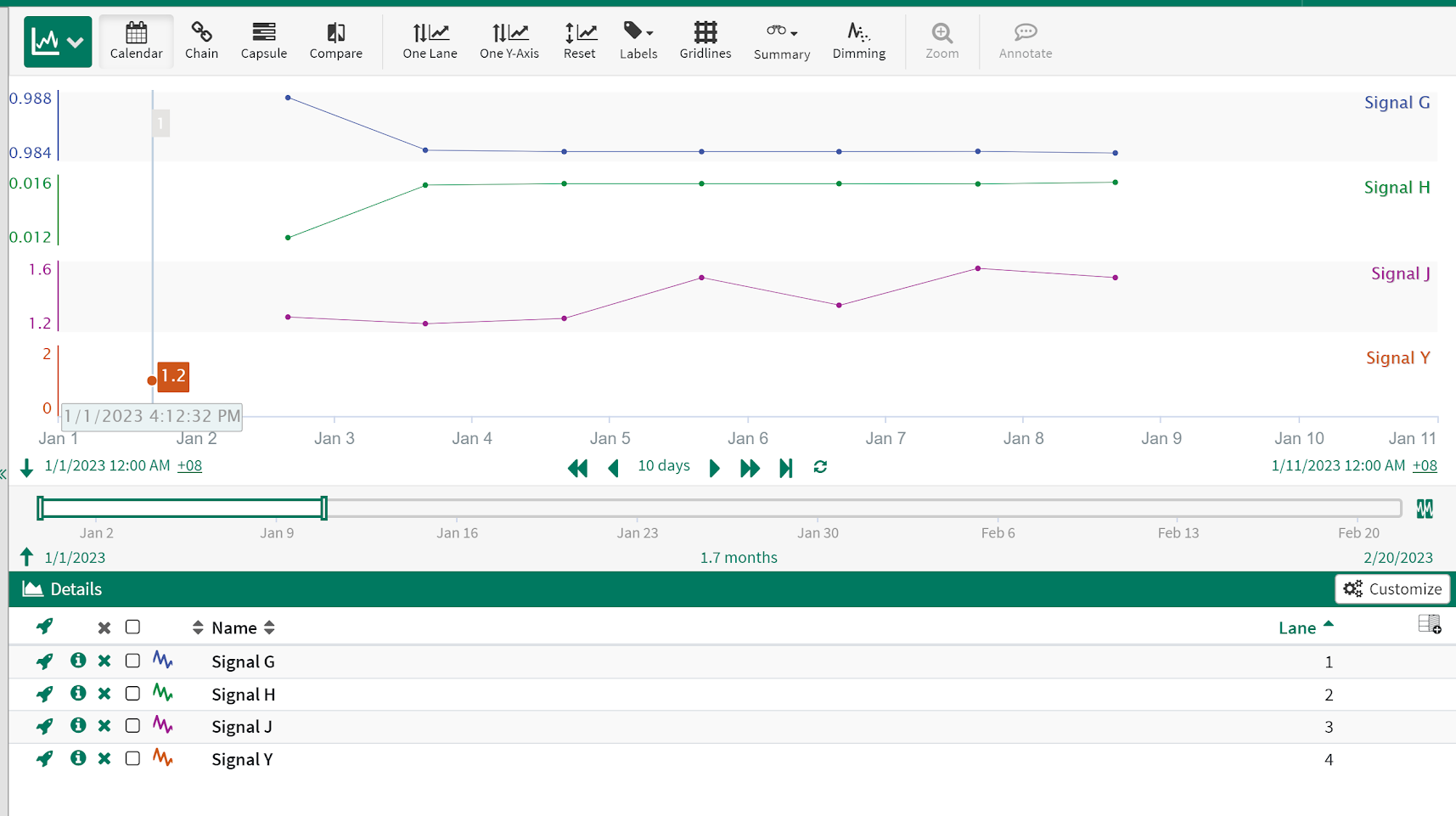Step 1: Set the start date and end date of the calculation.

```#Set the start date and end date of calculation
startdate = '2023-01-01'
enddate = '2023-01-09'```

Step 2: Set the workbook URL and workbook ID.

```#Set the workbook URL and workbook ID
workbook_url = 'workbook_url'
workbook_id = 'workbook_id'```

Step 3: Retrieve all raw data points for the time interval specified in Step 1 using spy.pull().

```#Retrieve all raw data points for the time internal specified in Step 1:
data = spy.pull(workbook_url, start = startdate, end = enddate, grid = None)
data```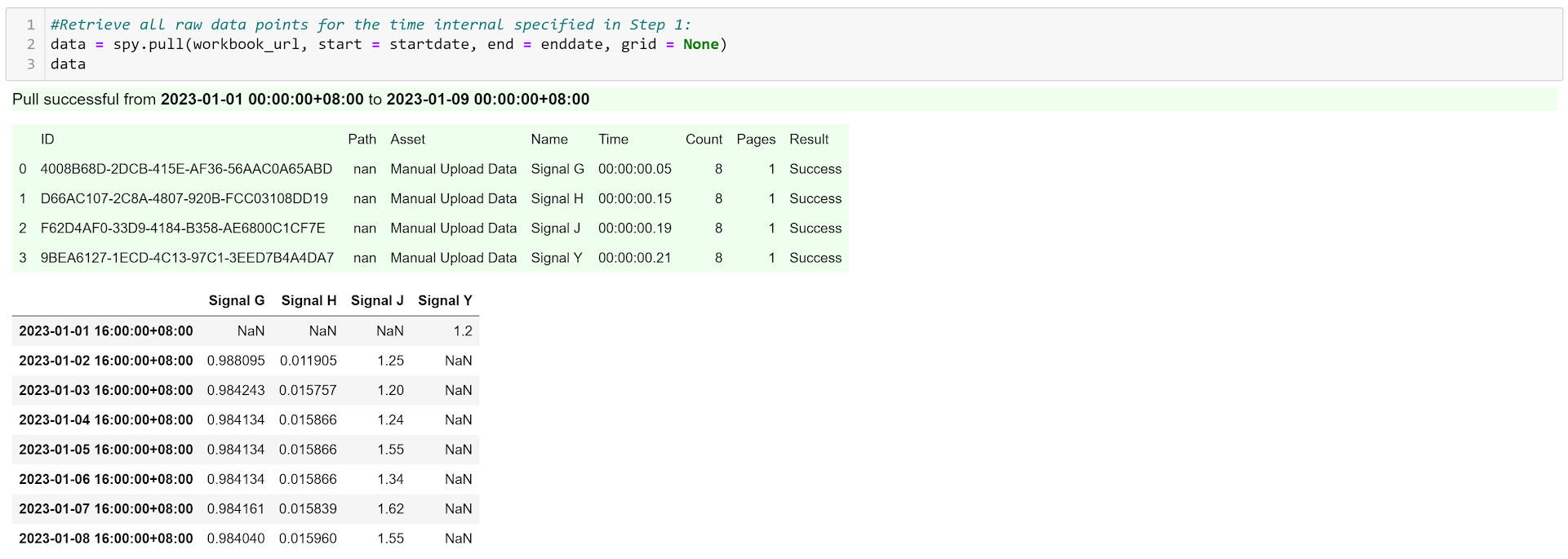Step 4: Calculate the value of Signal Y, (Yi = Gi * Y(i-1) + Hi * Ji)

```#Calculate the value of Signal Y (Yi = Gi * Y(i-1) + Hi * Ji)
for n in range(len(data)-1):
data['Signal Y'][n+1] = data['Signal G'][n+1] * data['Signal Y'][n] + data['Signal H'][n+1] * data['Signal J'][n+1]
data```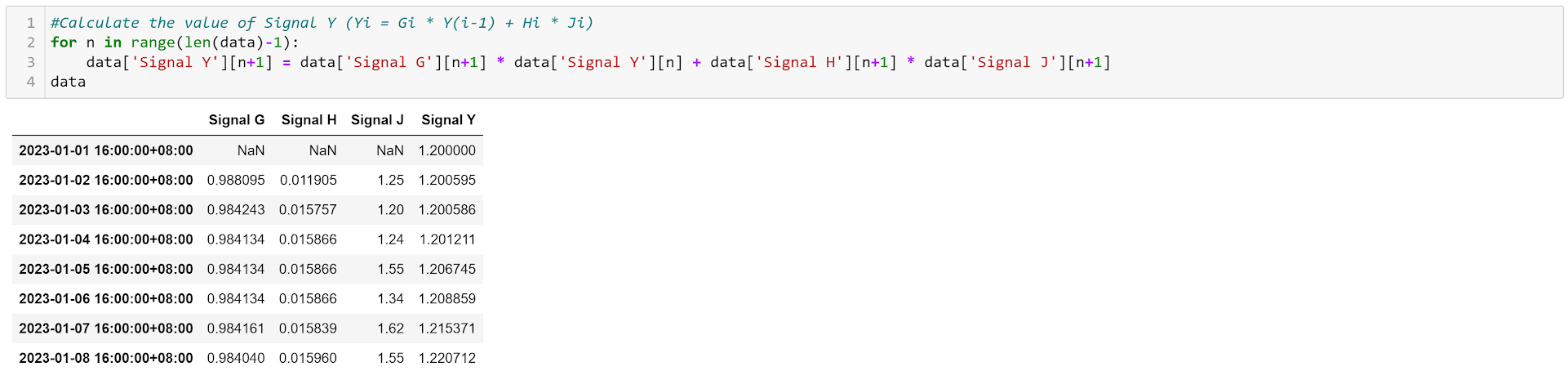Step 5: Push the calculated value of Signal Y to the source workbook using spy.push().

```#Push the calculated result of Signal Y to the source workbook
spy.push(data = data[['Signal Y']], workbook = workbook_id)```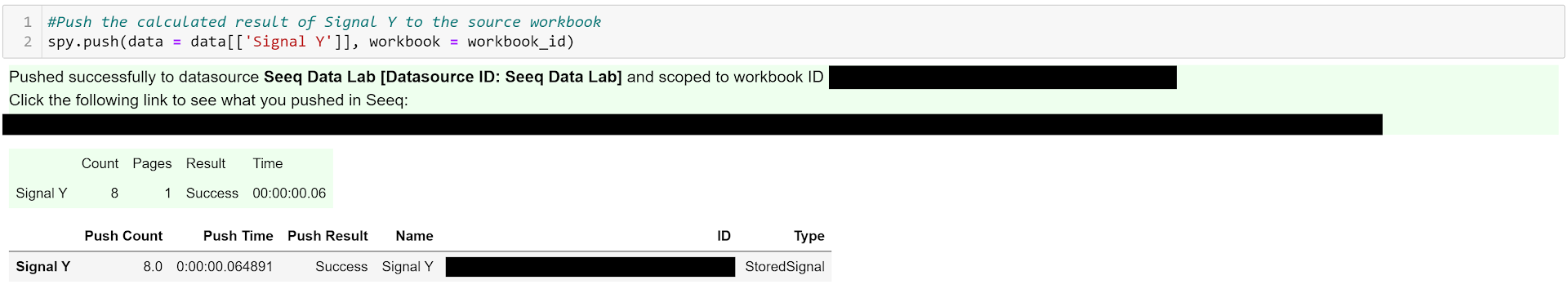•4
•3

## Create an account

Register a new account